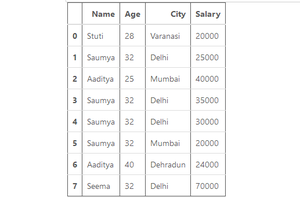# Get the data type of column in Pandas – Python

Let’s see how to get data types of columns in the pandas dataframe. First, Let’s create a pandas dataframe.

Example:

## Python3

 `# importing pandas library ` `import` `pandas as pd ` ` `  `# List of Tuples ` `employees ``=` `[ ` `            ``(``'Stuti'``, ``28``, ``'Varanasi'``, ``20000``), ` `            ``(``'Saumya'``, ``32``, ``'Delhi'``, ``25000``), ` `            ``(``'Aaditya'``, ``25``, ``'Mumbai'``, ``40000``), ` `            ``(``'Saumya'``, ``32``, ``'Delhi'``, ``35000``), ` `            ``(``'Saumya'``, ``32``, ``'Delhi'``, ``30000``), ` `            ``(``'Saumya'``, ``32``, ``'Mumbai'``, ``20000``), ` `            ``(``'Aaditya'``, ``40``, ``'Dehradun'``, ``24000``), ` `            ``(``'Seema'``, ``32``, ``'Delhi'``, ``70000``) ` `            ``] ` ` `  `  `  ` `  `# Create a DataFrame ` `df ``=` `pd.DataFrame(employees, ` `                  ``columns ``=` `[``'Name'``, ``'Age'``, ` `                             ``'City'``, ``'Salary'``]) ` `# show the dataframe ` `df`

Output:Dataframe

Method 1: Using Dataframe.dtypes attribute.

This attribute returns a Series with the data type of each column.

Syntax: DataFrame.dtypes.

Parameter: None.

Returns: dtype of each column.

Example 1: Get data types of all columns of a Dataframe.

## Python3

 `# importing pandas library ` `import` `pandas as pd ` ` `  `# List of Tuples ` `employees ``=` `[ ` `            ``(``'Stuti'``, ``28``, ``'Varanasi'``, ``20000``), ` ` ``           (``'Saumya'``, ``32``, ``'Delhi'``, ``25000``), ` ` ``           (``'Aaditya'``, ``25``, ``'Mumbai'``, ``40000``), ` ` ``           (``'Saumya'``, ``32``, ``'Delhi'``, ``35000``), ` ` ``           (``'Saumya'``, ``32``, ``'Delhi'``, ``30000``), ` ` ``           (``'Saumya'``, ``32``, ``'Mumbai'``, ``20000``), ` ` ``           (``'Aaditya'``, ``40``, ``'Dehradun'``, ``24000``), ` ` ``           (``'Seema'``, ``32``, ``'Delhi'``, ``70000``) ` ` ``           ] ` ` `  `  `  ` `  `# Create a DataFrame ` `df ``=` `pd.DataFrame(employees, ` `                  ``columns ``=` `[``'Name'``, ``'Age'``, ` `                             ``'City'``, ``'Salary'``]) ` ` `  `  `  ` `  `# Use Dataframe.dtypes to ` `# give the series of  ` `# data types as result ` `datatypes ``=` `df.dtypes ` ` `  `# Print the data types ` `# of each column ` `datatypes`

Output:

Example 2: Get the data type of single column in a Dataframe.

## Python3

 `#importing pandas library ` `import` `pandas as pd ` ` `  `# List of Tuples ` `employees ``=` `[(``'Stuti'``, ``28``, ``'Varanasi'``, ``20000``), ` ` ``           (``'Saumya'``, ``32``, ``'Delhi'``, ``25000``), ` ` ``           (``'Aaditya'``, ``25``, ``'Mumbai'``, ``40000``), ` ` ``           (``'Saumya'``, ``32``, ``'Delhi'``, ``35000``), ` ` ``           (``'Saumya'``, ``32``, ``'Delhi'``, ``30000``), ` ` ``           (``'Saumya'``, ``32``, ``'Mumbai'``, ``20000``), ` ` ``           (``'Aaditya'``, ``40``, ``'Dehradun'``, ``24000``), ` ` ``           (``'Seema'``, ``32``, ``'Delhi'``, ``70000``) ` ` ``           ] ` ` `  `# Create a DataFrame ` `df ``=` `pd.DataFrame(employees,  ` `                  ``columns ``=` `[``'Name'``, ``'Age'``, ` `                             ``'City'``, ``'Salary'``]) ` ` `  `# Use Dataframe.dtypes to give  ` `# data type of 'Salary' as result ` `datatypes ``=` `df.dtypes[``'Salary'``] ` ` `  `# Print the data types ` `# of single column ` `datatypes`

Output:

Method 2: Using Dataframe.info() method.

This method is used to get a concise summary of the dataframe like:

• Name of columns
• Data type of columns
• Rows in Dataframe
• non-null entries in each column
• It will also print column count, names and data types.

Syntax: DataFrame.info(verbose=None, buf=None, max_cols=None, memory_usage=None, null_counts=None)

Return: None and prints a summary of a DataFrame.

Example: Get data types of all columns of a Dataframe.

## Python3

 `# importing pandas library ` `import` `pandas as pd ` ` `  ` ``# List of Tuples ` `employees ``=` `[(``'Stuti'``, ``28``, ``'Varanasi'``, ``20000``), ` ` ``           (``'Saumya'``, ``32``, ``'Delhi'``, ``25000``), ` ` ``           (``'Aaditya'``, ``25``, ``'Mumbai'``, ``40000``), ` ` ``           (``'Saumya'``, ``32``, ``'Delhi'``, ``35000``), ` ` ``           (``'Saumya'``, ``32``, ``'Delhi'``, ``30000``), ` ` ``           (``'Saumya'``, ``32``, ``'Mumbai'``, ``20000``), ` ` ``           (``'Aaditya'``, ``40``, ``'Dehradun'``, ``24000``), ` ` ``           (``'Seema'``, ``32``, ``'Delhi'``, ``70000``) ` ` ``           ] ` ` `  `# Create a DataFrame ` `df ``=` `pd.DataFrame(employees, ` `                  ``columns ``=` `[``'Name'``, ``'Age'``,  ` `                             ``'City'``, ``'Salary'``]) ` ` `  `# Print complete details  ` `# about the data frame ` `df.info()`

Output:

My Personal Notes arrow_drop_upCheck out this Author's contributed articles.

If you like GeeksforGeeks and would like to contribute, you can also write an article using contribute.geeksforgeeks.org or mail your article to contribute@geeksforgeeks.org. See your article appearing on the GeeksforGeeks main page and help other Geeks.

Please Improve this article if you find anything incorrect by clicking on the "Improve Article" button below.

Article Tags :

Be the First to upvote.

Please write to us at contribute@geeksforgeeks.org to report any issue with the above content.